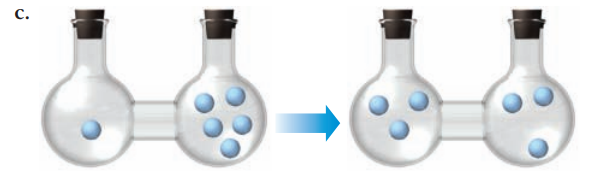×
Get Full Access to Chemistry: A Molecular Approach - 5 Edition - Chapter 19 - Problem 84
Get Full Access to Chemistry: A Molecular Approach - 5 Edition - Chapter 19 - Problem 84

×ISBN: 9780134874371 2047

## Solution for problem 84 Chapter 19

Chemistry: A Molecular Approach | 5th Edition

• Textbook Solutions
• 2901 Step-by-step solutions solved by professors and subject experts
• Get 24/7 help from StudySoup virtual teaching assistantsChemistry: A Molecular Approach | 5th Edition

4 5 1 346 Reviews
28
3
Problem 84

Determine the sign of $$\Delta S_{\text {sys }}$$ for each process.

a. dry ice subliming

b. dew formingText Transcription:

Delta S_sys

Step-by-Step Solution:
Step 1 of 3

Chemistry II Notes – Week 5 Ch. 16: Chemical Equilibrium  Dynamic equilibrium o Both sides of a chemical equation are happening at the same rate  Equilibrium constant o K o K is unitless o Ratio of products and reactants at equilibrium o Changing the concentration of anything changes everything o Law of mass action c d a b  K = [C] [D] / [A] [B]  aA+bB↔cC+dD o K is products over reactants o Reciprocal of reverse reaction  As product concentration increases, the reverse reaction rate increases o At equilibrium, concentrations are constant  Reactants and products aren’t necessarily equal in amount  There can be product or reactant favored reactions  When K is greater than one o Product favored reaction o Less reactants used  When K is less than one o Reactant favored reaction o Less product remaining  For gases o K p P xcP dd/ Paax P b o Partial pressures in atm o K p K xc(RT) Δn  Delta n is the change in moles between reactants and products  If it is zero then P will equal Kc  Heterogeneous Equilibrium o Liquids and+solids don-t count o H 2(l) ↔ H (g) + OH (g)  K c [H ][OH ] -

Step 2 of 3

Step 3 of 3

##### ISBN: 9780134874371

This full solution covers the following key subjects: . This expansive textbook survival guide covers 24 chapters, and 162 solutions. Chemistry: A Molecular Approach was written by Aimee Notetaker and is associated to the ISBN: 9780134874371. This textbook survival guide was created for the textbook: Chemistry: A Molecular Approach, edition: 5. The answer to “?Determine the sign of $$\Delta S_{\text {sys }}$$ for each process.a. dry ice sublimingb. dew forming Text Transcription:Delta S_sys” is broken down into a number of easy to follow steps, and 19 words. Since the solution to 84 from 19 chapter was answered, more than 201 students have viewed the full step-by-step answer. The full step-by-step solution to problem: 84 from chapter: 19 was answered by Aimee Notetaker, our top Chemistry solution expert on 06/03/22, 04:20PM.

## Discover and learn what students are asking

Calculus: Early Transcendental Functions : The Natural Logarithmic Function: Integration
?In Exercises 1-26, find the indefinite integral. $$\int \frac{1}{x-5} d x$$

Calculus: Early Transcendental Functions : Inverse Trigonometric Functions: Integration
?In Exercises 1-20, find the indefinite integral. $$\int \frac{1}{x \sqrt{1-(\ln x)^{2}}} d x$$

Chemistry: The Central Science : Electronic Structure of Atoms
?The radial probability function for a 2s orbital is shown here. Classify the fo

Unlock Textbook Solution